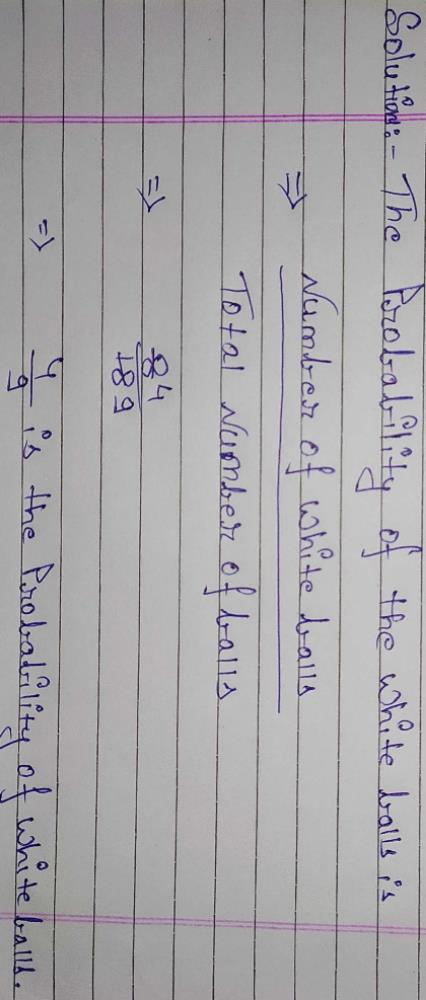Courses

# An urn contains 10 red and 8 white balls. One ball is drawn at random. The probability that the ball drawn is white isa)1/9b)2/9c)5/9d)4/9Correct answer is option 'D'. Can you explain this answer? Related Test: Test: Problems On Empirical Probability

## Class 9 Question

By Harish Chander · May 17, 2020 ·Class 9
Narinder Kaur answered May 17, 2020
Probability that the ball drawn is white = Number of white balls/Total number of balls
= 8/18
= 4/9

Avanish Jaiswal answered 3 weeks ago
Option D

Akshat Mishra answered Jun 06, 2020D) 4/9 is the correct answer

Vivek Pal answered 3 weeks ago

Ajay Mohta answered May 31, 2020
As we know:
10 red
8white
so total balls:
10+8=18
probability of white balls: white balls/ Total balls = 8/18
= 4/9
I hope you understood.

Shagun Bhatia answered May 18, 2020
Probability is
No. of desired outcome/No. of total outcomes
In this case it will be 8/18
When we reduce it to lowest form it becomes 4/9 (as 2 is a factor of both 8 and 18)
So the correct option is
D) 4/9

Aswath Amutha answered 2 weeks ago
4/9

Nitu Dutta answered May 26, 2020